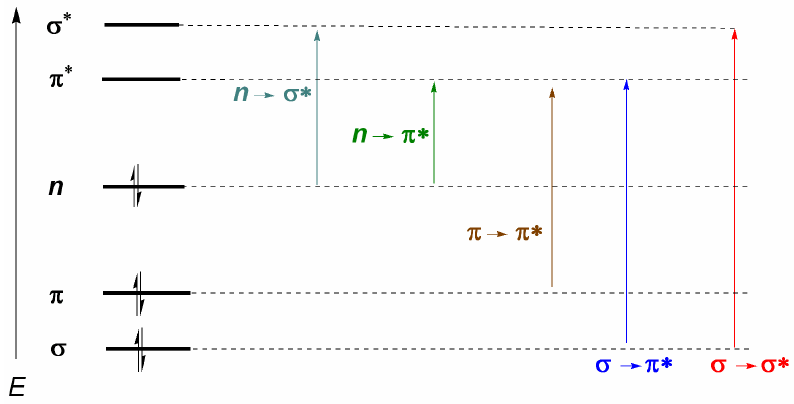Organic molecules distribute their electrons in different electronic levels called molecular orbitals. The lowest energy molecular orbitals are $\sigma$, followed by $\pi$ orbitals. When in the molecule there are atoms with free electronic pairs (oxygen, sulfur, nitrogen, halogens) we will have non-bonding levels n. These electronic levels are arranged in the bonding region of the diagram. In the antibonding region we find the molecular orbitals $\pi^{\ast}$ and $\sigma^{\ast}$. A molecule in its ground state has bonding and nonbonding orbitals occupied and antibonding orbitals unoccupied.

From what has been discussed above, it can be deduced that the electronic transitions must start from the bonding and nonbonding orbitals ending in the antibonding ones. Thus we can have the 5 types of electronic transitions shown in the following diagram.The most important electronic transition is usually from the HOMO (last occupied orbital) to the LUMO (first empty orbital) which corresponds to the smallest energetic jump and corresponds to a long wavelength.

In alkanes only $\sigma \rightarrow \sigma^{\ast}$ transitions are possible (ethane: $\lambda =135\;nm$). These bands cannot be observed with commercial spectrophotometers, since they scan from 200 nm. However, molecules that present conjugated double bonds present $\pi \rightarrow \pi^{\ast}$ transitions that can be observed (butadiene: $\lambda =217\;nm$).

When there are atoms with lone pairs in the molecule, the transition with the lowest energy (greatest wavelength) is $n\rightarrow \pi$. However, it is an unlikely transition, since there is no overlap between both orbitals, causing very weak bands. From this last comment the selection rule for UV-visible spectroscopy follows: $n\rightarrow \pi^{\ast}$ transitions are "forbidden". A low-probability transition is understood to be prohibited.